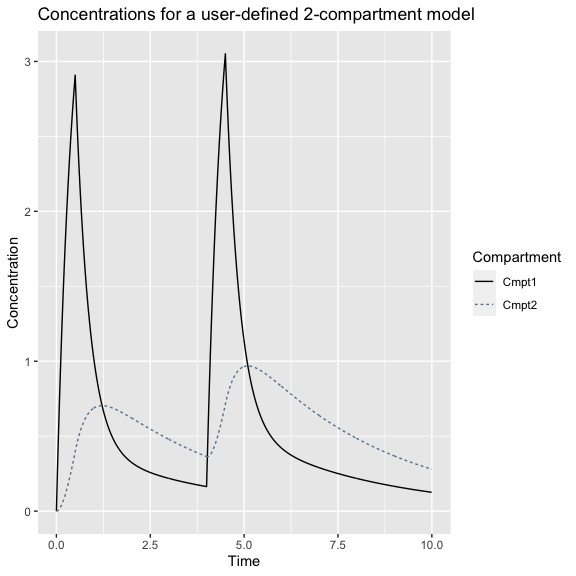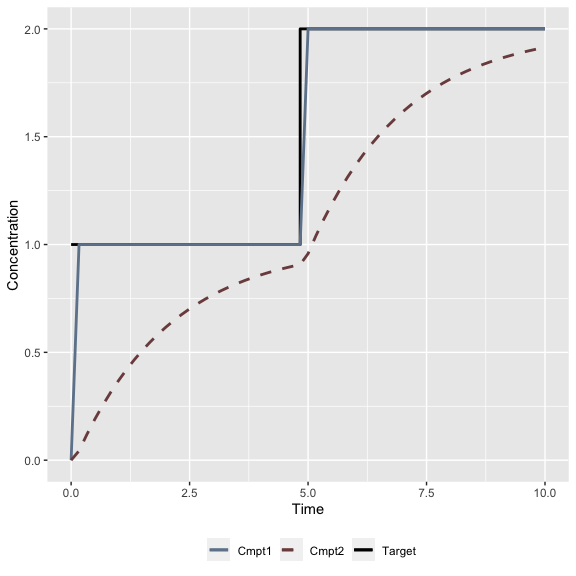# Simulation from a user-specified, ODE-based PK model

### User-defined PK functions using the tci package

The tci package provides closed-form solutions for 1-, 2-, 3-, and 3-compartment with effect-site PK models based on solutions described and code provided in Abuhelwa, Foster, and Upton (2015). It does not, however, include an ordinary differential equation (ODE) solver as many other R packages do, including mrgsolve, PKPDsim, and RxODE. tci package functions can nonetheless be applied to models from these packages through the creation of user-defined PK functions.

User-defined PK functions should be created to return concentrations at a vector of time points under a continuous infusion and should be assigned S3 class “pkmod.” To illustrate, we create an example two-compartment model based on ODEs is illustrated using the mrgsolve R package(Baron 2020). Using mrgsolve syntax, a model can be specified as a character string and compiled using the mcode function.

library(tci)
library(mrgsolve)
#>
#> Attaching package: 'mrgsolve'
#> The following object is masked from 'package:stats':
#>
#>     filter

# define two-compartment model based on ODEs
code <- '
$PARAM CL=10, V=10, Q = 10, V2=10$CMT A1 A2
\$ODE
dxdt_A1 = -(CL/V)*A1 - (Q/V)*A1 + (Q/V2)*A2;
dxdt_A2 = -(Q/V2)*A2 + (Q/V)*A1;
'
mod2cpt <- mcode("example", code)
#> Building example ...
#> done.

Initial parameter values are required by mrgsolve but can be updated as needed using the mrgsolve::update function. We now need to write a wrapper that takes as arguments a time at which to evaluate the PK model (tm), an infusion rate (kR), PK model parameters (pars), initial concentrations (init), and an infusion starting time (inittm). The starting time is used by functions in tci that iteratively calculate concentrations from a series of infusions. The function should return a matrix with concentrations for each compartment in the rows and evaluation times as columns.

# wrapper function to evaluate pk2 in format used by tci
pkmod2cpt_ode <- function(tm, kR, pars,
pkm = pk2,
init = c(0,0),
inittm = 0){

# begin times at 0 and end at last time evaluated
tm <- tm - inittm
end_inf <- max(tm)

# name parameter/initial concentrations
names(pars) <- toupper(names(pars))
names(init) <- c("A1","A2")

# pass parameters as list
pars <- sapply(pars, as.list)
vols <- unlist(pars[c("V","V2")])

# update parameters and initial values (as amounts)
mod2cpt <- update(mod2cpt, param = pars, init = init*vols)

# dosing regimen - mrgsolve function in terms of amount infused
event <- ev(amt =  kR*end_inf, time = 0, tinf = end_inf)

# simulate responses (skip tm=0 unless specified)
dat <- mrgsim_q(x = mod2cpt, # pk model
data = event, # dosing event
stime = tm) # evaluation times

# skip tm=0 unless specified in tm
dat <- dat@data[-1,]

# return concentrations with compartments in rows and times in columns
cons <- t(dat[,c("A1","A2")]) / vols

rownames(cons) <- colnames(cons) <- NULL
return(cons)
}
class(pkmod2cpt_ode) <- "pkmod"

For use with the tci package, the user-defined PK function should have class “pkmod.” We can now assign parameter values and predict from this model as we would any other.

# create dose
dose <- create_intvl(
as.matrix(cbind(time = c(0.5,4,4.5,10),
infrt = c(100,0,100,0)))
)

# model parameters
pars_2cpt <- c(CL = 15, V = 10, Q = 10, V2 = 20)

# predict concentrations
predict(pkmod2cpt_ode,
pars = pars_2cpt,
inf = dose,
tms = c(1,2,3))
#>      time        c1        c2
#> [1,]    1 1.0084483 0.6881986
#> [2,]    2 0.3252449 0.6216142
#> [3,]    3 0.2181003 0.4785318

# plot concentrations
plot(pkmod2cpt_ode,
pars = pars_2cpt,
inf = dose,
title = "Concentrations for a user-defined 2-compartment model")We can also apply TCI algorithms to calculate infusion schedules required to reach designated targets with the user-defined model.

# target concentration = 1 for times 0 - 5
# target concentration = 2 for times 5 - 10
tci_times <- c(0,5,10)
tci_targets <- c(1,2,2)

# plasma-targeting
inf_3cpt_plasma <- tci(Ct = tci_targets,
tms = tci_times,
pkmod = pkmod2cpt_ode,
pars = pars_2cpt,
tci_alg = "plasma")
# print infusion schedule
head(inf_3cpt_plasma)
#>         infrt     begin       end       dtm Ct c1_start   c2_start c1_end
#> [1,] 73.20009 0.0000000 0.1666667 0.1666667  1        0 0.00000000      1
#> [2,] 24.15371 0.1666667 0.3333333 0.1666667  1        1 0.04324996      1
#> [3,] 23.42262 0.3333333 0.5000000 0.1666667  1        1 0.11966300      1
#> [4,] 22.74993 0.5000000 0.6666667 0.1666667  1        1 0.18997314      1
#> [5,] 22.13097 0.6666667 0.8333333 0.1666667  1        1 0.25466780      1
#> [6,] 21.56144 0.8333333 1.0000000 0.1666667  1        1 0.31419547      1
#>          c2_end
#> [1,] 0.04324996
#> [2,] 0.11966300
#> [3,] 0.18997314
#> [4,] 0.25466780
#> [5,] 0.31419547
#> [6,] 0.36896883

# plot infusion schedule
plot(inf_3cpt_plasma)